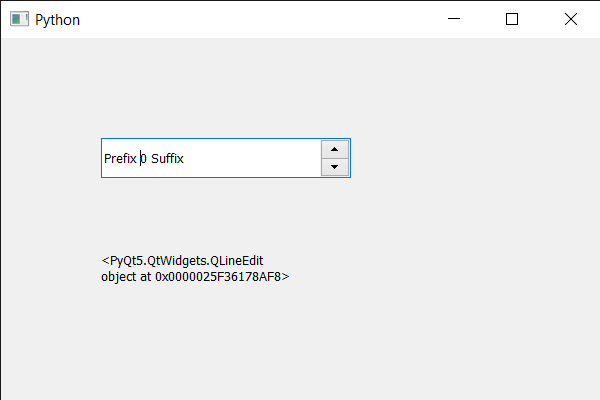# PyQt5 QSpinBox – Finding child using child type

• Last Updated : 19 May, 2020

In this article we will see how we can find the child of spin box using the child type, child type is the quality of child for example push button type is QPushButton. Spin box is made of two child one is line edit whose type is QLineEdit and other is those up and down button whose type are QValidator.

In order to do this we use findChild method

Attention geek! Strengthen your foundations with the Python Programming Foundation Course and learn the basics.

To begin with, your interview preparations Enhance your Data Structures concepts with the Python DS Course. And to begin with your Machine Learning Journey, join the Machine Learning - Basic Level Course

Syntax : spin_box.findChild(child_type)

Argument : It takes child type as argument

Return : It returns the child object, if no child is found it return None

Below is the implementation

 `# importing libraries``from` `PyQt5.QtWidgets ``import` `*` `from` `PyQt5 ``import` `QtCore, QtGui``from` `PyQt5.QtGui ``import` `*` `from` `PyQt5.QtCore ``import` `*` `import` `sys`` ` ` ` `class` `Window(QMainWindow):`` ` `    ``def` `__init__(``self``):``        ``super``().__init__()`` ` `        ``# setting title``        ``self``.setWindowTitle(``"Python "``)`` ` `        ``# setting geometry``        ``self``.setGeometry(``100``, ``100``, ``600``, ``400``)`` ` `        ``# calling method``        ``self``.UiComponents()`` ` `        ``# showing all the widgets``        ``self``.show()`` ` `        ``# method for widgets``    ``def` `UiComponents(``self``):``        ``# creating spin box``        ``self``.spin ``=` `QSpinBox(``self``)`` ` `        ``# setting geometry to spin box``        ``self``.spin.setGeometry(``100``, ``100``, ``250``, ``40``)`` ` `        ``# setting range to the spin box``        ``self``.spin.setRange(``0``, ``999999``)`` ` `        ``# setting prefix to spin``        ``self``.spin.setPrefix(``"Prefix "``)`` ` `        ``# setting suffix to spin``        ``self``.spin.setSuffix(``" Suffix"``)`` ` ` ` `        ``# getting child using child type``        ``child ``=` `self``.spin.findChild(QLineEdit)`` ` `        ``# creating a label``        ``label ``=` `QLabel(``self``)`` ` `        ``# making it multi line``        ``label.setWordWrap(``True``)`` ` `        ``# setting its geometry``        ``label.setGeometry(``100``, ``200``, ``200``, ``60``)`` ` `        ``# setting text to the label``        ``label.setText(``str``(child))`` ` ` ` ` ` `# create pyqt5 app``App ``=` `QApplication(sys.argv)`` ` `# create the instance of our Window``window ``=` `Window()`` ` `# start the app``sys.exit(App.``exec``())`

Output :My Personal Notes arrow_drop_up